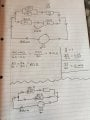#### djsfantasi

Joined Apr 11, 2010
8,672
What have you done so far to solve the problem? Please show your work so we can understand where you get stuck, your understanding of the circuit and how we can help.

There are some very simple first steps you can take to simplify the diagram. What are two 40Ω resistors in parallel equivalent to? For example…

#### Blake Harrison

Joined Nov 29, 2014
2Thankyou for the quick response. I'v read through the information in the link, sorry if I haven't posted this correctly I will make sure to show my working out and provide more information in any future posts.

I'm unsure whether the working out I have been doing is the correct method but I have attached a photo, trust me there's a lot more screwed up pieces of paper too. I'v just started the electrical principles course and I'm finding it hard to wrap my head around even the most simple methods, that's the reason I'v created an account on this site to try and get somebody else's perspective see if it helps.

The first thing I'v attempted to do is calculate the resistors in parallel which was R1R2 / R1 + R2. Then add the resistors in series R3 + R4.
Then I did V/R=I for each and rewrite the circuit simplified with the new information I have.

Struggling where to go next.

#### djsfantasi

Joined Apr 11, 2010
8,672
Good work... You are very close.

This forum' e-textbook has a chapter on series and parallel circuits.
What does it tell you about the voltage across the 60 Ω branch and the (20+R) Ω branch?

Once you have the voltage, since you know the resistance in one branch, you can use Ohm's law.

Show where this get's you. [Edit: Oh, you did already!]

Your calculation using the 20 Ω value is incomplete; you need to account for the value of R, and we don't know it yet.
But, your calculation of the current through the 60 Ω branch is correct (personally, I'd have rounded up to 0.417)
So what does that tell you? How can this information be used to calculate R?

#### WBahn

Joined Mar 31, 2012
27,893
You are making one of the classic mistakes when you use Ohm's Law to calculate the current through the 20Ω resistance because Ohm's Law requires that you use the voltage across THAT resistance. You used 25V, but 25V is NOT the voltage across THAT resistance, it is the voltage across THAT resistance PLUS the voltage across the unknown resistance.

Think about the problem in terms of what you know, what you want to know, and what you need to know.

You want to know the unknown resistance.

To find the unknown resistance, you need to know the current flowing in it and the voltage appearing across it. So treat those as two new problems and see if you can solve them.

To find the current flowing in the unknown resistance, you need to know the total current flowing out of the battery and the total current flowing in all of the other branches. You know the total current since it is given, so now you need to find the current flowing through all of the other branches (and there is only one of them, namely the branch with the two 30Ω resistors).

Do you have the information you need to find the current in the 30Ω resistors? You need the total resistance of that branch and the total voltage across that branch. Do you have (or can you calculate) those two pieces of information?

To find the voltage across the unknown resistor, you need to know the voltage across the branch containing it and the voltage across the other components in that branch. So your new problem is to find the voltage across the two 40Ω resistors. To solve that problem, you need to know the current flowing through them and the equivalent resistance of them. If you've already solved the prior problems, do you have this information (or can you calculate it).